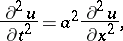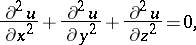# Mathematical physics

(diff) ← Older revision | Latest revision (diff) | Newer revision → (diff)
Jump to: navigation, search

The theory of mathematical models of physical events; it holds a special position, both in mathematics and physics, being found at the junction of the two sciences.

Mathematical physics is closely connected with the part of physics concerned with the construction of mathematical models and, at the same time, is a branch of mathematics, since the methods of investigation of these models are mathematical. Included in the notion of methods of mathematical physics are those mathematical methods which are used for the construction and study of mathematical models describing large classes of physical phenomena.

The methods of mathematical physics, as also the theory of mathematical models in physics, were first intensively developed by I. Newton in the creation of the foundations of classical mechanics, universal gravitation and the theory of light (cf. Newton laws of mechanics). Subsequent development of the methods of mathematical physics and their application to the study of mathematical models in an extensive domain of physical phenomena is connected with the names of J.L. Lagrange, L. Euler, J. Fourier, C.F. Gauss, B. Riemann, M.V. Ostrogradski, and many other scholars. A major contribution to the development of the methods of mathematical physics was made by A.M. Lyapunov and V.A. Steklov.

Beginning in the second half of the 19th century, the methods of mathematical physics were successfully applied in the study of mathematical models of physical phenomena connected with the various physical field and wave processes in electrodynamics, acoustics, elasticity theory (cf. Elasticity, mathematical theory of), hydro- and aerodynamics (cf. Hydrodynamics, mathematical problems in), and a number of other research directions in the physics of continuous media. The mathematical models of this class of phenomena are most frequently described by partial differential equations, which have been given the name "the equations of mathematical physics" (cf. Mathematical physics, equations of).

In addition to the differential equations of mathematical physics, in describing the mathematical models of physics one finds applications of integral equations and integro-differential equations, variational and probability-theoretical methods, potential theory, methods from complex function theory and from a number of other branches of mathematics. In connection with the vigorous development of computational mathematics, direct numerical methods, using computers (finite-difference methods and other computational algorithms for boundary value problems), acquire a special significance for the investigation of mathematical models of physics, and, by the methods of mathematical physics, have allowed the effective solution of new problems in gas dynamics (cf. Gas dynamics, numerical methods of), transport theory (cf. Transport equations, numerical methods), plasma physics, including the inverse problems for these most important directions of physical research.

Theoretical research in quantum physics (cf. Quantum field theory) and relativity theory, the widespread use of computers in various areas of mathematical physics, including inverse (ill-posed) problems, have required a significant expansion of the arsenal of mathematical methods used in mathematical physics. Side by side with the traditional branches of mathematics there has been widespread application of operator theory, distribution theory, the theory of functions of several complex variables, and topological and algebraic methods. This intensive interaction of mathematical physics, mathematics and the use of computers in scientific research has led to a significant expansion of the subject, the creation of new classes of models and has raised modern mathematical physics to a new level. It has made a great contribution to the acceleration of scientific and technological progress.

The formulation of problems in mathematical physics results in the construction of mathematical models which describe the fundamental laws of the class of physical phenomena being studied. The formulation consists of deriving the equations (differential, integral, integro-differential, or algebraic) satisfied by the quantities characterizing the physical process. Here one proceeds from fundamental laws of physics, taking into account only the more essential features of the phenomenon and ignoring a number of secondary characteristics. Such laws are the usual laws of conservation of, for example, momentum, energy, the number of particles, etc. They are connected with symmetry groups, spatio-temporal ones and others. This leads to the fact that for the description of processes of different physical natures — but having common characteristic features — the same mathematical model can be used. For example, the mathematical problems for the simplest equation of hyperbolic type,obtained originally by J. d'Alembert (1747) for the description of the free oscillations of a homogeneous string, turned out to be applicable also for the description of a broad range of wave processes in acoustics, hydrodynamics and other areas of physics. Similarly, the equationthe boundary value problems of which were originally studied by P.S. Laplace (at the end of the 18th century) in connection with the construction of a gravitation theory (cf. Laplace equation), has subsequently found application in the solution of many problems in electrostatics, elasticity theory, the problem of steady motion of an ideal fluid, etc. Each mathematical model of physics corresponds to a whole class of physics processes.

It is also characteristic of mathematical physics that many general methods used for the solution of the problems of mathematical physics were developed from particular methods of solution of concrete physical problems and, in their original form, did not have a rigorous mathematical foundation and sufficient perfection. This applies to such well-known methods of solution of problems in mathematical physics as the methods of Ritz and Galerkin (cf. Galerkin method; Ritz method), the methods of perturbation theory, the Fourier transform, and many others, including the method of separation of variables (cf. Separation of variables, method of). The effectiveness of the application of all of these methods for the solution of concrete problems is one of the reasons for giving them a mathematical proof and generalizations, leading in a number of cases to the appearance of new directions in mathematics.

The influence of mathematical physics on the various branches of mathematics is shown by the fact that the development of mathematical physics, reflecting the needs of the natural sciences and practical needs, has, on occasions, implied a re-orientation of the directions of research in certain already-established domains of mathematics. The posing of problems in mathematical physics connected with the use of mathematical models of real physical phenomena has led to a change in the fundamental problems considered in the theory of partial differential equations. The theory of boundary value problems (cf. Boundary value problem, partial differential equations) has arisen, subsequently allowing a connection between differential equations, integral equations and variational methods.

The study of mathematical models of physics by mathematical methods not only allows one to obtain quantitative characteristics of physical phenomena and to compute, with a given degree of accuracy, the course of real processes, but also provides the possibility of deep penetration into the very essence of the physical phenomena, an explanation of hidden laws and the prediction of new effects. The trend towards a more detailed study of physical phenomena leads to more complicated mathematical models describing these phenomena, which in turn make it impossible to apply analytic methods of research for these models. This is explained, in particular, by the fact that the mathematical models of real physical process are, as a rule, non-linear, that is, are described by non-linear equations of mathematical physics. For a detailed investigation of these models direct numerical methods using computers are successful. For typical problems in mathematical physics, the application of numerical methods leads, e.g., to replacing the equations of mathematical physics, for functions of continuous arguments, by algebraic equations for grid functions, given on a discrete set of points (a grid). In other words, instead of a continuous model of a medium one introduces a discrete analogue. The application of numerical methods in a number of cases allows one to replace complex, time-consuming and expensive physical experiments by a significantly more economical mathematical (numerical) experiment. A mathematical experiment, properly carried out, is a basis for a choice of optimal conditions for a real physical experiment, the choice of parameters of complicated physical equipment, the determination of the conditions for the appearance of new physical effects, etc. Thus, numerical experiments usually expand the domain of effective utilization of mathematical models of physics phenomena.

Mathematical models of physics phenomena, as with all models, need not convey all the aspects of a phenomenon. Establishing the adequacy of an accepted model for investigating a phenomenon is possible only with the help of a practical criterion: comparing the results of theoretical research with experimental data.

In many cases it is possible to judge the adequacy of a model on the basis of the solution of an inverse problem of mathematical physics, when inferences on those properties of the phenomena which are inaccessible to direct observation are made on the basis of their indirect physical and observable effects.

A characteristic feature of mathematical physics is to construct mathematical models which not only describe and explain the already established physical phenomena in the domain of events being studied, but also allow one to predict phenomena not already discovered. The classical example of such a model is Newton's theory of universal gravitation, which not only unified the motion of the then known bodies of the solar system, but also permitted the prediction of the existence of new planets. On the other hand, new experimental data need not always fit into the accepted model. To take account of this a refinement of the model may be required.

How to Cite This Entry:
Mathematical physics. Encyclopedia of Mathematics. URL: http://encyclopediaofmath.org/index.php?title=Mathematical_physics&oldid=15675
This article was adapted from an original article by A.N. TikhonovA.A. SamarskiiA.G. Sveshnikov (originator), which appeared in Encyclopedia of Mathematics - ISBN 1402006098. See original article